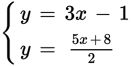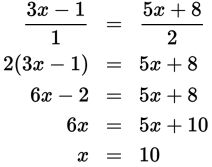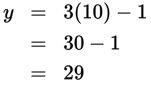# SAT Math Multiple Choice Question 340: Answer and Explanation

### Test Information

Question: 340

10.If (x, y) represents the solution to the system of equations shown above, what is the value of y?

• A. 10
• B. 19
• C. 29
• D. 31

Explanation:

C

Difficulty: Medium

Category: Heart of Algebra / Systems of Linear Equations

Strategic Advice: When a system consists of two equations already written in terms of y, the quickest way to solve the system is to set the equations equal to each other and then use inverse operations.

Getting to the Answer: Don't let the fraction intimidate you-you can write the first equation as a fraction over 1 and use cross-multiplication.Don't let A fool you-the question is asking for the value of y, not the value of x. To find y, substitute 10 for x in either equation and simplify: